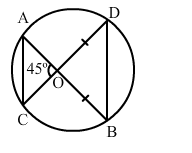# In the given figure, O is the point of intersection of two chords AB and CD such

Question:

In the given figure, O is the point of intersection of two chords AB and CD such that OB = OD and ∠AOC = 45°. Then, ∆OAC and ∆ODB are(a) equilateral and similar
(b) equilateral but not similar
(c) isosceles and similar
(d) isosceles but not similar

Solution:

(c) isosceles and similar

In ∆AOC and ∆ODB, we have:

$\angle A O C=\angle D O B \quad$ (Vertically opposite angles)

and $\angle O A C=\angle O D B \quad$ (Angles in the same segment)

Therefore, by AA similarity theorem, we conclude that $\triangle A O C \sim \triangle D O B$.

$\Rightarrow \frac{O C}{O B}=\frac{O A}{O D}=\frac{A C}{B D}$

Now, $O B=O D$

$\Rightarrow \frac{O C}{O A}=\frac{O B}{O D}=1$

$\Rightarrow O C=O A$

Hence, $\triangle \mathrm{OAC}$ and $\triangle \mathrm{ODB}$ are isosceles and similar.